Latest Banking jobs   »   Quantitative Aptitude Quiz For ESIC- UDC,...

# Quantitative Aptitude Quiz For ESIC- UDC, Steno, MTS Prelims 2022-20th January

Q1. Mixture P and mixture Q have wine and water in ratio 3 : 7 and 1 : 4 respectively. At what ratio both mixture must mixed to get a ratio of wine to water 1 : 3 ?
(a) 2:3
(b) 1:1
(c) 2:1
(d) 1:2
(e) None of these

Q2. If profit obtained on selling an article is equal to % above the C.P. at which the article sold then find C.P (in Rs)?
(a) None of these
(b) 200
(c) 300
(d) Cannot be determined
(e) 100

Q3. In a test who score above 40% is passed. Rahul gives the test then what is the probability that he failed.
(a)1/6
(b)2/5
(c)1/4
(d) 1/2
(e)2/3

Q4. Ram and Shyam together can do a work in 16 days. Shyam takes twice time as Ram takes to do the same work. In how many days Shyam alone can complete that work.
(a) 16 days
(b) 32 days
(c) 24 days
(d) 40 days
(e) 48 days

Q5. Average of four numbers is increased by 1 when a number is replaced by x and average of that four number is decreased by 1 when an another number is replaced with x. What is the difference of the both numbers?
(a) 6
(b) 8
(c) 16
(d) 4
(e) Cannot be determined

Q6. P, Q and R together can do a work in 27 days. They together work for 17 days and after that R left the work and the remaining work is done by P and Q together in 16 ⅔ days. Work done by P in 4 days is equal to work done by R in 3 days. Then, Find time taken by P alone to do this work ?
(a) 90 days
(b) 80 days
(c) 100 days
(d) 95 days
(e) 105 days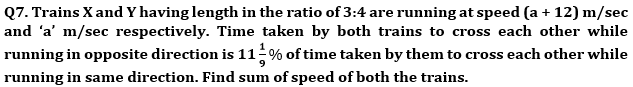(a) 116 m/sec
(b) 96 m/sec
(c) 108 m/sec
(d) 86 m/sec
(e) 85 m/sec

Q8. Rajat invested Rs 6,000 at rate of interest 20% per annum. The interest was compounded yearly for the first year and in the second year it was compounded half yearly. What will be the total interest earned at the end of the second year?
(a) Rs 2924
(b) Rs 2784
(c) Rs 2824
(d) Rs 2624
(e) Rs 2712

Q9. A shopkeeper sold an article at a profit of 1/8 th of its SP to a person. If shopkeeper sold it at 25% more than previous selling price it will have Rs 68 more as profit. Find its selling price.
(a) Rs. 280
(b) Rs. 272
(c) Rs. 290
(d) Rs. 300
(e) Rs. 264

Q10. Two vessels contain liquid A and liquid B in the ratio 3:7 and 3:2 respectively. In what ratio should the contents of these two vessels be mixed such that the final ratio of liquid B and liquid A in the resultant solution becomes 23:17.
(a) 5 : 3
(b) 4 : 3
(c) 6 : 5
(d) 7 : 5
(e) 7 : 4

Q11. Sumit can swim 12 km upstream and 16 km downstream in 5 hours. If ratio of speed of stream to that of speed of Sumit in upstream is 1 : 2, then find the speed of Sumit in still water.
(a) 6 km/hr
(b) 5 km/hr
(c) 4 km/hr
(d) 8 km/hr
(e) 7 km/hr

Q12. Ravi invested 20% less than Sikha and Sikha invested 25% more than Mohit. The ratio of time period of investment of Ravi, Sikha and Mohit is 3: 4: 5 respectively and the sum of profit shares of Ravi and Mohit is Rs1600. Then find the profit share of Sikha.
(a) Rs. 1000
(b) Rs. 1050
(c) Rs. 1200
(d) Rs. 1150
(e) Rs. 950

Q13. Ratio of first and second number is 9:10 and ratio of first and third number is 3:4. Find difference of second and third number if average of all the three numbers is 62?
(a) 8
(b) 10
(c) 12
(d) 14
(e) 16

Q14. Ratio of marked price to cost price of an item is 5 : 3. Discount percent given on selling that item which is equal to the profit percent earned on that item. Find marked price is what percent of selling price of that item ?
(a) 120%
(b) 125%
(c) 133 ⅓%
(d) 140%
(e) 150%

Q15. Radius of smaller circle is four-fifth of radius of larger circle. Difference of area of these two circle is 182 cm² less than twice of area of a square having side equal to radius of smaller circle. Find circumference of bigger circle ?
(a) 352 cm
(b) 176 cm
(c) 220 cm
(d) 264 cm
(e) 308 cm

Solutions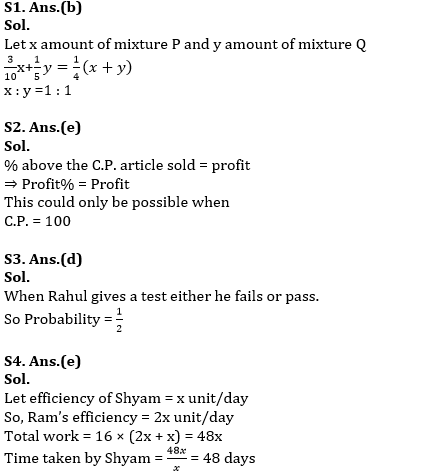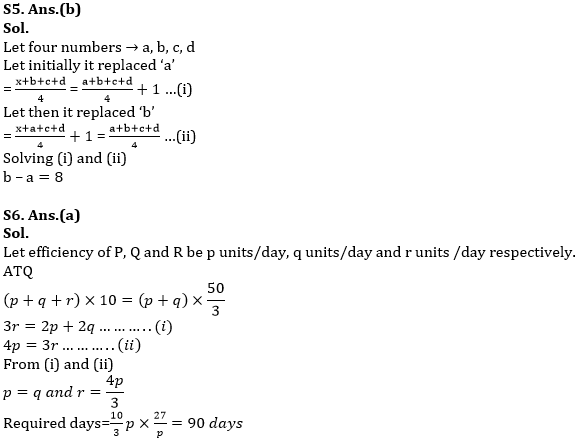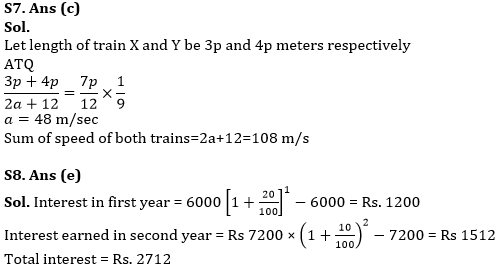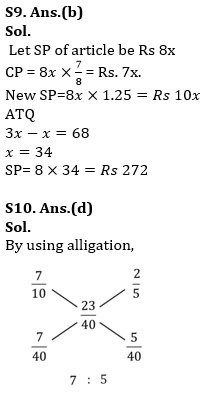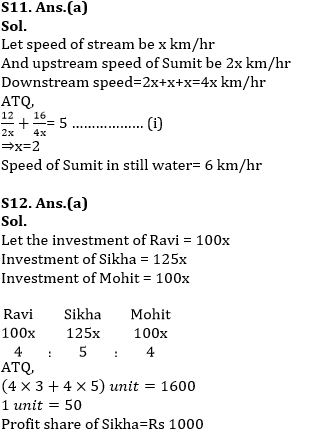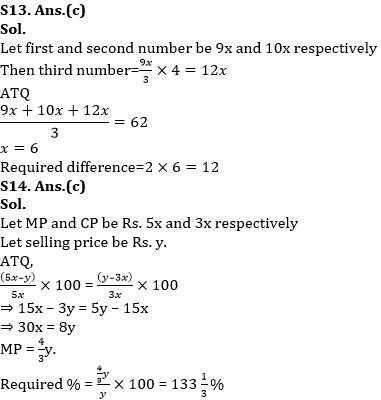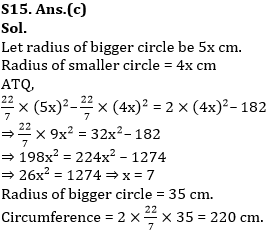#### Congratulations!